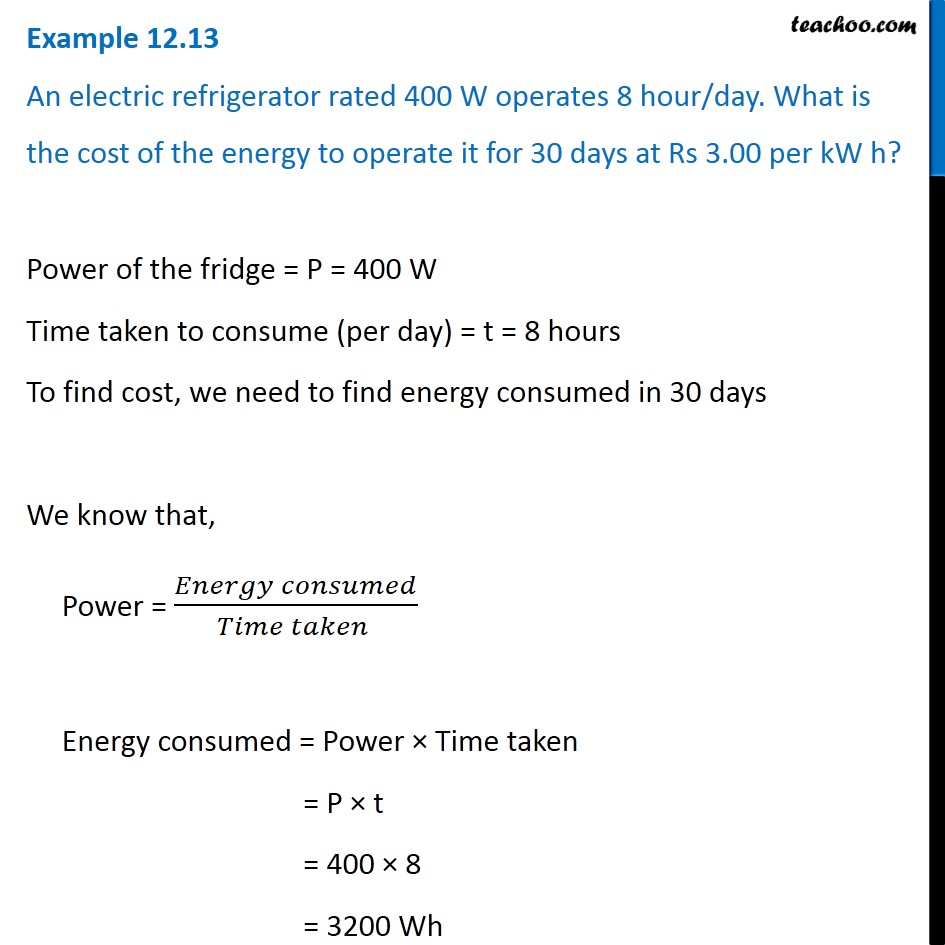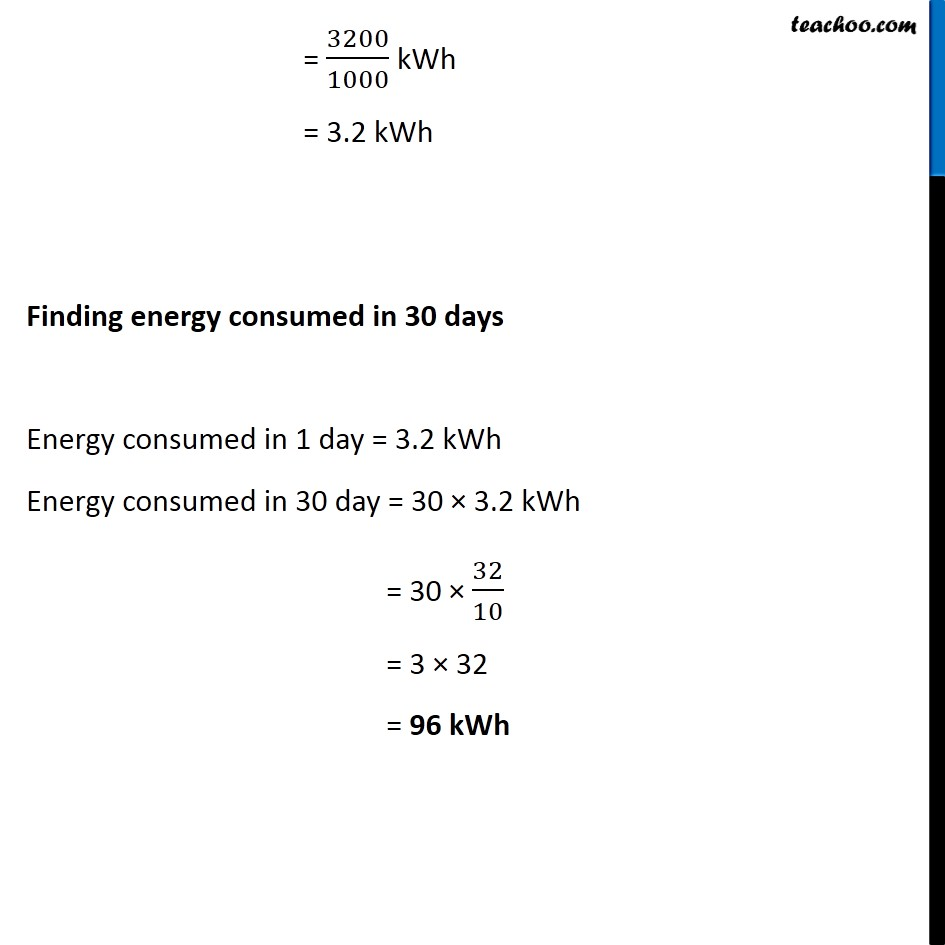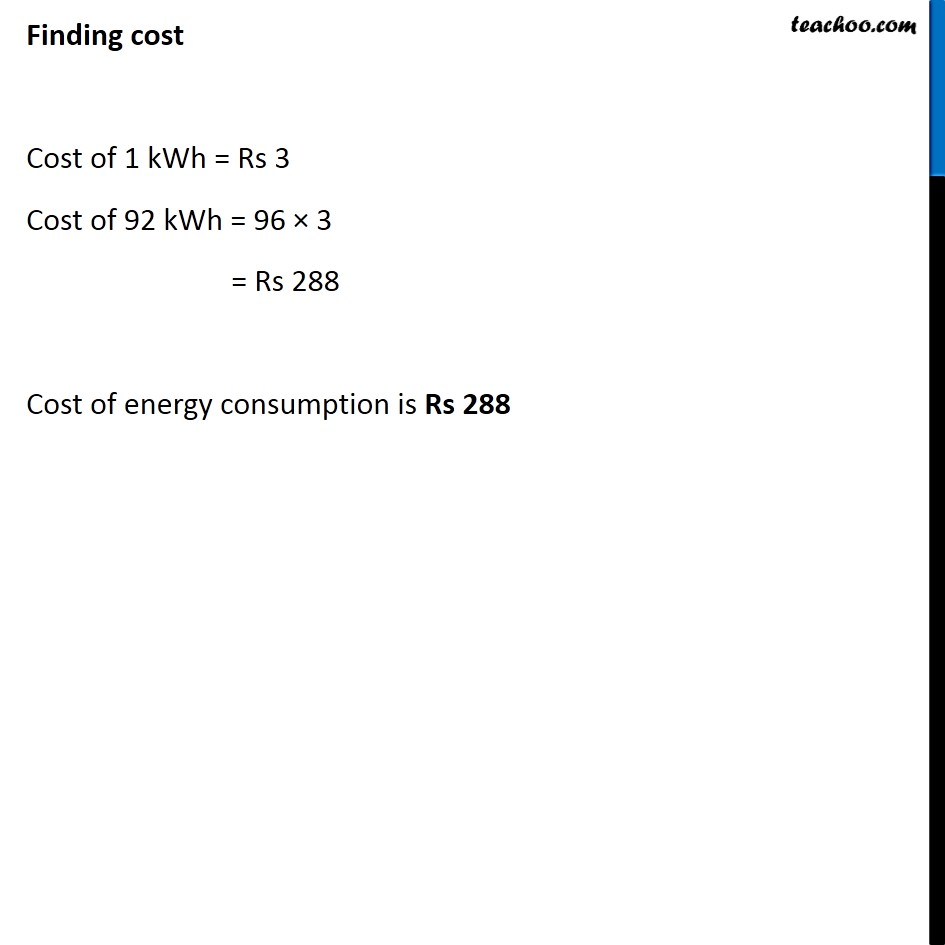Examples from NCERT Book

Class 10
Chapter 12 Class 10 - Electricity

## An electric refrigerator rated 400 W operates 8 hour/day. What is the cost of the energy to operate it for 30 days at Rs 3.00 per kW h?Learn in your speed, with individual attention - Teachoo Maths 1-on-1 Class

### Transcript

Example 12.13 An electric refrigerator rated 400 W operates 8 hour/day. What is the cost of the energy to operate it for 30 days at Rs 3.00 per kW h? Power of the fridge = P = 400 W Time taken to consume (per day) = t = 8 hours To find cost, we need to find energy consumed in 30 days We know that, Power = (𝐸𝑛𝑒𝑟𝑔𝑦 𝑐𝑜𝑛𝑠𝑢𝑚𝑒𝑑)/(𝑇𝑖𝑚𝑒 𝑡𝑎𝑘𝑒𝑛 ) Energy consumed = Power × Time taken = P × t = 400 × 8 = 3200 Wh = 3200/1000 kWh = 3.2 kWh Finding energy consumed in 30 days Energy consumed in 1 day = 3.2 kWh Energy consumed in 30 day = 30 × 3.2 kWh = 30 × 32/10 = 3 × 32 = 96 kWh Finding cost Cost of 1 kWh = Rs 3 Cost of 92 kWh = 96 × 3 = Rs 288 Cost of energy consumption is Rs 288